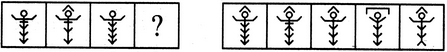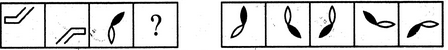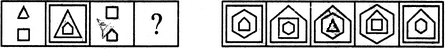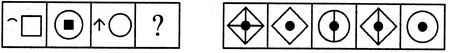# Non Verbal Reasoning - Analogy

### Exercise :: Analogy - Section 1

Each of the following questions consists of two sets of figures. Figures A, B, C and D constitute the Problem Set while figures 1, 2, 3, 4 and 5 constitute the Answer Set. There is a definite relationship between figures A and B. Establish a similar relationship between figures C and D by selecting a suitable figure from the Answer Set that would replace the question mark (?) in fig. (D).

36.

Select a suitable figure from the Answer Figures that would replace the question mark (?).(A)     (B)      (C)     (D)                  (1)      (2)      (3)      (4)      (5)

 A. 1 B. 2 C. 3 D. 4 E. 5

Explanation:

One of the arrowheads moves from the lower part to the upper part of the figure above the circle and gets vertically inverted.

37.

Select a suitable figure from the Answer Figures that would replace the question mark (?).(A)     (B)      (C)     (D)                  (1)      (2)      (3)      (4)      (5)

 A. 1 B. 2 C. 3 D. 4 E. 5

Explanation:

The figure rotates through 180o.

38.

Select a suitable figure from the Answer Figures that would replace the question mark (?).(A)     (B)      (C)     (D)                  (1)      (2)      (3)      (4)      (5)

 A. 1 B. 2 C. 3 D. 4 E. 5

Explanation:

The lower element gets enlarged and becomes the outermost element; the upper element gets slightly enlarged and becomes the middle element and a new element with one more number of sides than the lower element appears as the innermost element.

39.

Select a suitable figure from the Answer Figures that would replace the question mark (?).(A)     (B)      (C)     (D)                  (1)      (2)      (3)      (4)      (5)

 A. 1 B. 2 C. 3 D. 4 E. 5

Explanation:

The LHS element becomes a closed figure by combining with three similar elements. The RHS element gets reduced in size, turns black and moves to the centre of the other figure.

40.

Select a suitable figure from the Answer Figures that would replace the question mark (?).(A)     (B)      (C)     (D)                  (1)      (2)      (3)      (4)      (5)

 A. 1 B. 2 C. 3 D. 4 E. 5